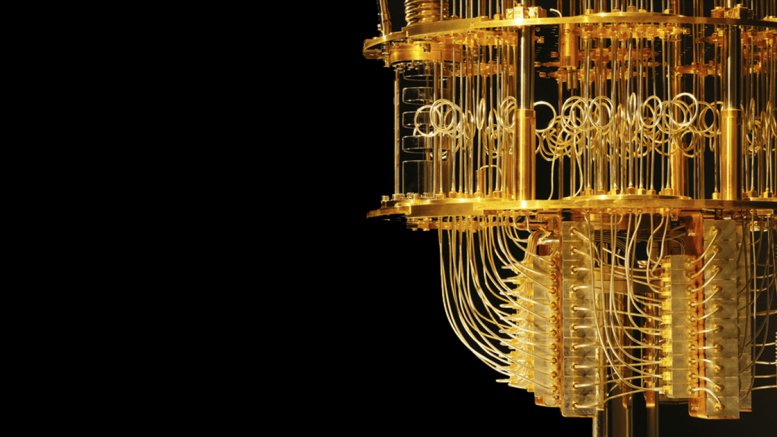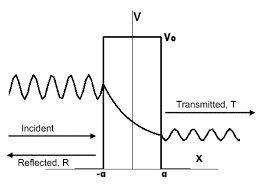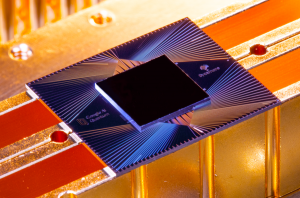# The Future of ComputingQuantum computing proposes massive advancements to traditional supercomputers in certain computational fields | Source: IBM

In the modern era, global economic markets are dominated by computationally savvy players, with increasingly intricate data analysis techniques commanding the major decisions of multinational corporations. Although many of these algorithms are written by teams of data scientists, the heavier workloads are often tasked to massive supercomputers capable of carrying out intensive computational tasks. These supercomputers can be applied to fields such as weather forecasting, economic market predictions, molecular modeling, as well as physical simulations for mathematically complex situations (such as subatomic particle interactions).

In order to increase the efficiency of microchips, manufacturers compete to decrease the size of the transistors within these silicon semiconductor chips. By decreasing the size, the subsequent distance for electrons to travel also decreases, resulting in faster execution times for each boolean operation. On May 11, 2021, IBM unveiled a two-nanometer (2nm) chip, the smallest semiconductor process on the market. However, as these computers progress, there exists one fatal drawback to advancements in traditional computers and supercomputers: quantum mechanics.

As these transistors decrease in size, the quantum properties of the electrons in question begin to affect the system, as the particle can undergo quantum tunneling. This tunneling effect is a result of the wave function (a mathematical function describing the probability amplitude which relates the location of a particle at a given point in space with its corresponding energy value) propagating through a theoretical barrier. In the context of minimizing the size of semiconductors, the transistor acts as the barrier with the electron having the energy potential to tunnel past it. Because of this tunneling phenomenon, the logic operations comprising every computer algorithm become unreliable. So, despite the incremental improvements to chip efficiency, there exists a natural physical limit to the extent of this manufacturing.A diagram depicting the wave function $$\Psi(x,t)$$ describing an electron tunneling through a potential barrier derived by solving the Schrödinger equation analytically. The standard process to find the solution involves defining three stages of the wave function. The pre-barrier wave acts as a sinusoid or complex exponential $$(\psi_{\text{I}}(x) =Ae^{ikx}+Be^{-ikx})$$, the internal-barrier wave drops off exponentially $$\psi_{\text{II}}(x)=Ce^{\beta x}+De^{\beta x})$$, and the final wave function takes a smaller amplitude complex exponential $$(\psi_{\text{III}}(x)=Fe^{ikx}+Ge^{-ikx})$$. | Source: MIT

Unlike their traditional counterparts, quantum computers make use of this uncertainty, utilizing the superposition of a collection of entangled subatomic particles to carry out the computational process. While normal computers store information in units called bits (valued at either a $$1$$ or a $$0$$, corresponding to either a true or false state in boolean logic), quantum computers store information in qubits. These qubits similarly reflect a $$1$$ or a $$0$$ when observed, but until this moment of observation, each qubit exists in a state of superposition — both a $$1$$ and $$0$$ at the same time. In order to read the final state of these particles after observation, directional values for a quantum property known as spin are assigned to be respective $$1$$ or $$0$$ values. This state of superposition between $$|1 \rangle$$ and $$|0 \rangle$$ eigenstates allows for numerous computational paths to be taken simultaneously within the quantum computer, decreasing the processing time and resulting in solutions to complex tasks that would take a traditional computer millions of years.

The randomness associated with subatomic particles (and ergo, qubits) also makes it difficult to obtain a working algorithm that can operate as predicted. However, a phenomenon dubbed “quantum entanglement” allows for each of the qubits to correlate their measurements with each other, resulting in a system of informationally dependent and correlated particles. Entanglement occurs when quantum particles share a certain spatial proximity or are directed to interact in a specific manner. By knowing the value of one entangled qubit, the value of other entangled qubits can also be determined, as they exist in reference to one another. One major goal for physicists is to increase the total number of qubits in a given quantum computer, without them falling out of entanglement. If this occurs, the interference from a disentangled qubit will offset results from the quantum computer as a whole, resulting in unreliable data.

In October 2019, Google announced that they had achieved quantum supremacy (a theoretical demonstration that a given programmable quantum computer can solve a complex problem that is unsolvable by any classical computer within any amount of time). After working with a team of Stanford researchers, Google developed a quantum chip composed of 54 qubits named “Sycamore.” According to the company, the quantum computer had performed such a computationally impossible task (by classical standards) that no traditional system could achieve the same solution within a reasonable amount of time. However, competitors in the field such as IBM, D-Wave, and Azure protested the proclaimed feat, for the result achieved by Sycamore would simply take a classical supercomputer approximately 10,000 years, and therefore does not satisfy the condition of impossibility dictated by quantum supremacy.Google’s most functional (in practice) quantum computer Sycamore with a total number of 54 qubits — the topic of immense controversy among quantum computing giants | Source: The New York Times

As of 2021, Google has retracted its statement of quantum supremacy and continues to work on developing systems containing more and more stable entangled qubits. They currently hold the market record for the largest number yet with Bristlecone,” a 72 qubit chip, as competitors in the technology industry including Intel and Microsoft also strive to be the first to achieve true quantum supremacy.

The technology industry continues to strive for progressively superior and efficient data analysis, making massive leaps in each company’s respective fields. As this competition accelerates, so do the advancements inside and outside the sector, making changes across international markets. The future of computing is ever-changing.